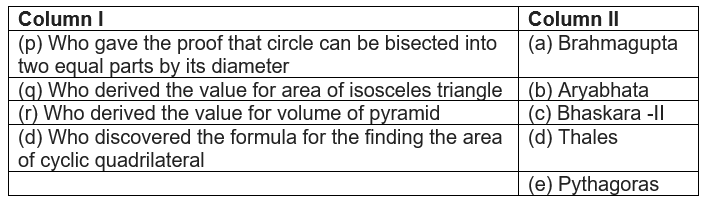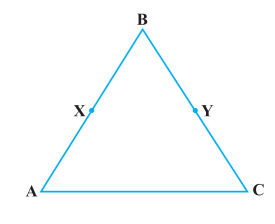# Euclids geometry class 9 extra questions

Given below are the Class 9 Maths Extra Questions for Euclid Geometry
a. Concepts questions
b. Calculation problems
c. Multiple choice questions
e. Fill in the blanks

Question 1.
Which of this statement are axioms, postulates, definition, Theorem?
1. If equals are added to equals, the wholes are equal
2. The edges of a surface are lines
3. All right angles are equal to one another
4. If first thing is greater than second and second is greater than third, then first is greater than third
5. The whole is greater than the part
6. Two distinct lines cannot have more than one point in common
7. For every line l and for every point P not lying on the line l, there exists a unique line m passing through P and Parallel to l
Solution
Axioms:  (a), (d), (e), (g)
Postulates: (c)
Definition: (b)
Theorem: (f)

Question 2
Define each of the following terms
a. Parallel lines
b. Angle
c. Line segment
e. Square
Solution
a. Two lines are parallel when they are not intersecting and are coplanar
b. Angle is the figure formed by two rays with common initial points
c. A line segments is a part of line with two end points.
d. The distance from the centre of the circle to any point on the circle is called radius of the circle
e. A square is a quadrilateral of which all the four angles are rights angles and four sides are equal
f. A simple closed figure made up of four lines

Question 3
True or False statement
a. In geometry, we take point, line and plane as undefined term
b. Two lines drawn in a plane always intersect at a point
c. Only one line can pass through points X and Y
d. If two circle are equal, there radii is also equal
e. Three points are concurrent if they have only one common point
f. The statements that are proved are called postulates
g. The whole is greater than the part is a postulate
h. If the area of square X equals to the area of rectangle Y and the area of rectangle Y is equal to area of circle Z,then area of square X equals to area of circle Z
Solution
1. True
2. False, as parallel lines never intersect
3. True
4. True
5. True
6. False
7. False
8. True

## Multiple choice Questions

Question 4.
Euclid belongs to
a. Greece
b. Italy
c. Germany
d. Egypt
Solution
(a)

Question 5.
The total number of propositions in the elements is?
a. 20
b. 7
c.13
d. 465
Solution
(d)

Question 6.
Ram salary is equal to Mohan salary, Due to recession, the salaries of ram and Mohan are made half. The final salary of Ram will still be equal to Mohan. This is are per
a. Ist axiom
b. 7Th axiom
c. 6th axiom
d. 2nd axiom
Solution
(b)

Question 7.
Which of these statements are false?
a.The number of dimensions in the solid is three
b. The number of dimensions in the surface is two
c. The number of dimensions of a point is 1
d. None of these
Solution
(c)

Question 8.
The number of line segments determined by the three non collinear points is
a. one
b. two
c. three
d. four
Solution
c

Question 9.

Boundaries of the solid are
a. lines
b. points
c. surface
d. curves
Solution c

Question 10.
It is known that if $p+q=11$ then $p+q+r=11+r$. The Euclid axioms that illustrates this statement is
a. Ist axiom
b.3Th  axiom
c. 4th axiom
d. 2nd axiom
Solution
(d)

## Match the column

Question 11.Question 12.In the above figure we have
$\text{BX} =\frac {1}{2} \text{AB}$
$\text {BY} = \frac {1}{2} \text {BC}$
and AB = BC. Show that
BX = BY
Solution c
we have
$\text {BY} = \frac {1}{2} \text {BC}$
Now AB=BC
Therefore
$\text {BY} = \frac {1}{2} \text {AB}$
Now from Euclid Axiom "Things which are halves of the same things are equal to one another"
BX=BY

Question 13.
Read the following two statements which are taken as axioms:
(i) If two lines intersect each other, then the vertically opposite angles are not equal.
(ii) If a ray stands on a line, then the sum of two adjacent angles, so formed is equal to 180°
Solution
We know that when two lines intersect each other, vertically opposite angles are equal and this is theorem. So (i) is incorrect
(ii) is an axiom as we know already about. So Statement is false and no axiom and statement II is true and axiom.
So this is not consistent pair of axioms

Question 14.
(i) Things which are equal to the same thing are equal to one another.
(ii) If equals are added to equals, the wholes are equal.
(iii) Things which are double of the same thing are equal to one another.
Check whether the given system of axioms is consistent or inconsistent
Solution
Some of the Euclids axioms are
(a)Things which are equal to same things are equal to one another
(b) If equals are added to equals, the wholes are equal
(c) If equals are subtracted from equals, the remainders are equal
(d)Things which are double of the same things are equal to one another
(e) Things which are halves of the same things are equal to one another.
So clearly statement (i) ,(ii) and (iii) are axoims .Since they cannot be deduce any from others, These axioms are consistent

## Summary

This Euclids geometry class 9 extra questions is prepared keeping in mind the latest syllabus of CBSE . This has been designed in a way to improve the academic performance of the students. If you find mistakes , please do provide the feedback on the mail.

• Notes
• NCERT Solutions
• Assignments
• Revision sheet